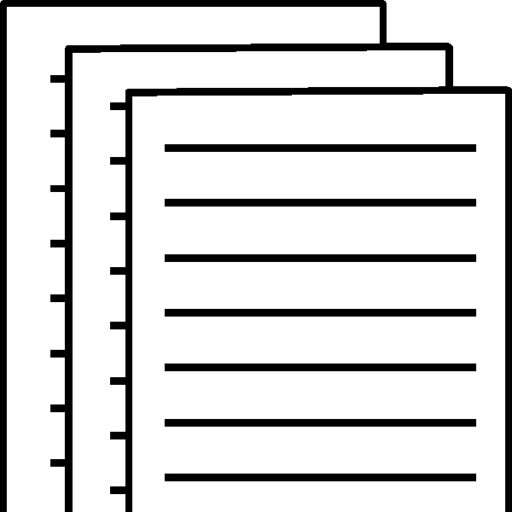## FIN 501 Module 3 – SLP Capital Budgeting and the Cost of Capital

 CAPITAL BUDGETING AND THE COST OF CAPITAL

## Find out your chosen company’s credit rating. Rating agencies such as Moody’s and Standard and Poor’s assign ratings to companies. AAA is high, AA is lower, BBB is even lower, etc. The higher the rating, the lower the cost of debt capital. Explain what your company’s credit rating is and the reasons for the high or low rating based on your research. Also, use the Fidelity Fixed Income Web page to find out what the current return is for a 30-year bond for a corporation with the rating that your company has. This yield will be the approximate cost of debt capital for your company. We will call the cost of debt RD.

Rating = AAA

30-year bond = RD = 4.22%

## Now estimate the cost of equity for your company. First you will need the beta; you already found this for your Module 1 SLP. You will also need the three-month treasury bill yield, which we will use as our measure of the risk-free rate. This rate should be listed on the Fidelity Fixed Income Web page linked above. Finally, you will need the equity risk premium. You can find estimates of this on many Web pages including Fidelity Fixed Income or Gutenberg Research. It is usually around 5%. Once you have this information, you can estimate the cost of equity as the 30-year treasury bill yield rate plus beta multiplied by the equity premium:

Beta = 1.04

3mo. U.S. Treasury bill = 2.05%

Cost of Equity = risk-free rate + Beta * (Equity Premium)
Cost of Equity = RE = 2.05% + 1.04 * 4.42% = 6.6468%

Show your calculations. We will call the cost of equity RE.

## Now find out how much of the firm’s capital is equity and how much is debt. For the total value, look at the balance sheet for your company as found on Google Finance or a similar Web page. The total value of your company will be “total liabilities and shareholder’s equity.” The proportion of debt will be total liabilities divided by total value, which we will call D/V. The proportion of equity will be shareholder’s equity divided by total value, or E/V. If you calculate them correctly, the proportions will add up to one.

Total Liabilities & Stockholders’ Equity = \$249,097

D/V = Total Liabilities / Total Value
D/V = \$159,450/\$249,097 = 64.01%

E/V = Shareholder’s Equity / Total Value
E/V = \$89,647 / \$249,097 = 35.99%

D/V + E/V = 64.01% + 35.99% = 100%

## Now we have all the information we need to get at least a rough ballpark estimate of WACC. Let’s assume a corporate tax rate of 35%. So, the formula we will use is WACC = (E/V)* RE +(D/V)* RD *(1-.35)

WACC = (E/V)* RE +(D/V)* RD *(1-.35)
WACC = 35.99% * 6.6468% + 64.01% * 4.22% * (1-.35) = 4.15%
WACC = 35.99% * 6.6468% + 64.01% * 4.22% * (1-.25) = 4.42%
WACC = 35.99% * 6.6468% + 64.01% * 4.22% * (1-.10) = 4.82%

According to Cost of Capital by Sector:

These numbers are higher than my calculations above even if I reduce the tax rate.

• Software (Systems & Application) = 7.32%
• Software (Internet) = 8.36%
• Software (Entertainment) = 6.70%

To view and download a complete answer, scroll down to the bottom to pay